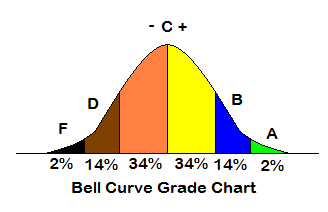Total Test Population:
Highest Score:
Lowest Score:
Fill in the total population of people that took a test, then fill in the highest test score achieved and the lowest test score achieved of that population.

## How do you grade a test when you grade on a curve?

A Normal Bell Curve calculates a grading curve from a comparison of student results. A Bell Curve will calculate grades based on the amount of standard deviations from the mean average.

Note: A small test population with a minimal difference between the highest score and the lowest score will not result in an effective Bell Curve scale.

Using a Bell Curve, the population grades will break down as follows:

Example: In a true Bell Curve grading method, a test population of 100 students should have 2 students with a grade of "A", 14 students with a "B", a total of 68 with a "C", 14 with a "D"
and 2 with a grade of "F".